# MongoDB 常用查询操作

06/16 10:24MongoDB 查询操作可实现大部分关系型数据库的常用查询操作，本文对 MongoDB 常用查询进行讲解。

# 条件大小比较操作

\$eq 查询与条件值相等的文档，类似关系型数据库的 =
\$ne 查询与条件值不相等或不存在的文档，类似关系型数据库的 !=
\$gt 查询大于条件值的文档，类似关系型数据库的 >
\$gte 查询大于或等于条件值的文档，类似关系型数据库的 >=
\$lt 查询小于条件值的文档，类似关系型数据库的 <
\$lte 查询小于或等于条件值的文档，类似关系型数据库的 <=
\$in 查询 \$in 数据里值的文档，类似关系型数据库的 in
\$nin 与 \$in 查询相反，类似关系型数据库的 not in

``````db.article.find({"visitor": {\$gte:150}})
``````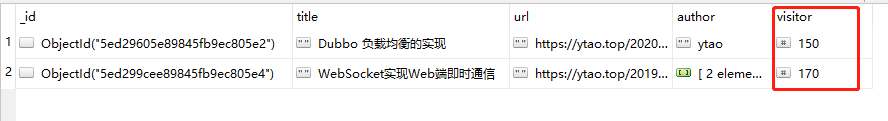``````db.article.find({"visitor": {\$in:[70, 150]}})
``````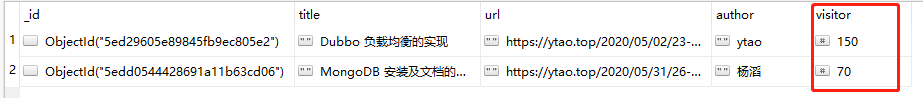# 逻辑操作符

\$and 表示所有条件同时满足时成立
\$nor `\$and`相反，所有条件都不满足时成立
\$or 只要有一个条件满足则成立
\$not 表示字段存在并且不符合条件

`\$and` 查询`author=ytao``visitor=150`的文档

``````db.article.find(
{\$and:[
{"author":{\$eq:"ytao"}},
{"visitor":{\$eq:150}}
]}
)
``````

`\$nor`查询不是`author=ytao`和不是`visitor=170`的文档

``````db.article.find(
{\$nor:[
{"author":{\$eq:"ytao"}},
{"visitor":{\$eq:170}}
]}
)
``````

`\$or`查询`author=ytao``visitor=170`的文档

``````db.article.find(
{\$or:[
{"author":{\$eq:"ytao"}},
{"visitor":{\$eq:170}}
]}
)
``````

`\$not`查询不是`author=ytao`的文档

``````db.article.find(
{"author":{\$not:{\$eq:"ytao"}}}
)
``````

# 元素操作符

\$exists 判断文档中字段是否存在，`true`为存在，`false`为不存在
\$type 筛选指定字段类型的文档

`\$exists`查询`author`字段存在的文档

``````db.article.find(
{"author":{\$exists:true}}
)
``````

`\$type`查询`author`字段为数组的文档

``````db.article.find(
{"author":{\$type:"array"}}
)
``````

# 正则表达式

MongoDB 支持正则表达式匹配文档，通过正则表达我们可以实现关系型数据库的模糊查询，以及更加强大匹配规则，其使用语法有三种：

``````{ < field >: { \$regex: /pattern/, \$ options ： '<options>' } }
{ < field >: { \$regex: 'pattern', \$ options ： '<options>' } }
{ < field >: { \$regex: /pattern/<options> } }
``````

options 选项 说明
i 匹配过程忽略大小写
x 匹配过程忽略空格
m 匹配多行数据，但都是从每行的起点和结尾匹配
s 将多行转换成一行后进行匹配，可匹配换行符`\n`字符串

``````db.article.find(
{"author":{\$regex:/Tao/, \$options:'i'}}
)
``````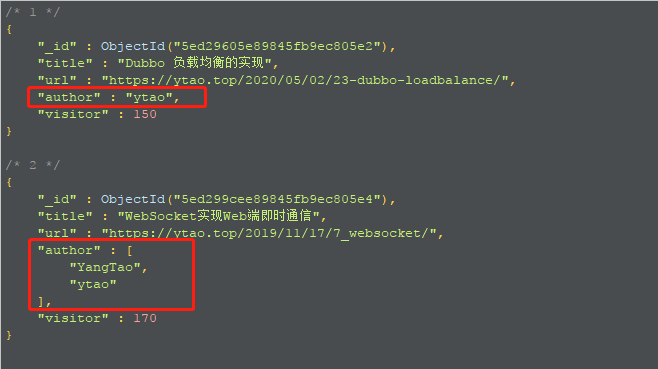# 聚合操作

``````db.collection.aggregate([
{聚合操作一},
{聚合操作二}
])
``````

## 条件筛选

\$match 用来进行条件筛选，可以使用一些条件限制来进行查询。

``````db.article.aggregate([
{ \$match: <条件> }
])
``````

``````db.article.aggregate([
{ \$match: {
\$and: [
{"author": {\$eq: "ytao"}},
{"visitor": {\$gt: 100}}
]}
}
])
``````

## 分组操作

\$group 是分组操作符，类似于关系型数据库中的`group by`操作。其语法格式为：

``````db.collection.aggregate([
{
\$group:{
"_id":"\$<分组字段名>",
<显示结果的字段名称>:{<运算符>:"\$<运算符计算的字段名>"}
}
}
])
``````

\$avg 当前组的平均数
\$sum 当前组的总和
\$min 当前组的最小值
\$max 当前组的最大值
\$first 当前组的第一个的值
\$last 当前组的最后一个的值
\$push 数组形式展示指定的当前组字段值

``````db.article.aggregate([
{
\$group:{
"_id":"\$author",
"avg_visitor":{\$sum:"\$visitor"}
}
}
])
``````

## 字段显示

``````db.collection.aggregate([
{
\$project:{
"<字段名>": <0或1>,
"<字段名>":<0或1>
}
}
])
``````

``````db.article.aggregate([
{
\$project:{
"_id": 0,
"title": 1,
"visitor": 1
}
}
])
``````

``````db.collection.aggregate([
{
\$project:{
"<字段名>": {<操作符>: <条件>},
"<字段名>": {<操作符>: <条件>},
}
}
])
``````

``````db.article.aggregate([
{
\$project:{
"titleField":{ \$toUpper:"\$title" }
}
}
])
``````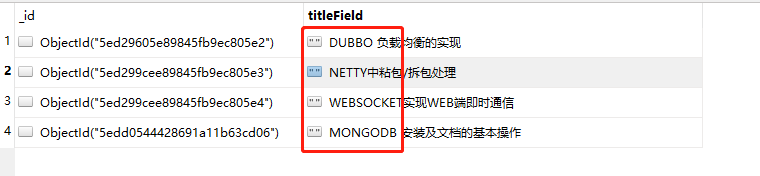## 排序操作

\$sort是文档排序操作符，类似关系型数据中的`order by`指令。`\$sort`排序用`1``-1`表示正序和倒序。

``````db.collection.aggregate([
{
\$sort:{
"<排序字段名>": <1 或 -1>
}
}
])
``````

`visitor`字段名进行倒序排序：

``````db.article.aggregate([
{
\$sort:{
"visitor": -1
}
}
])
``````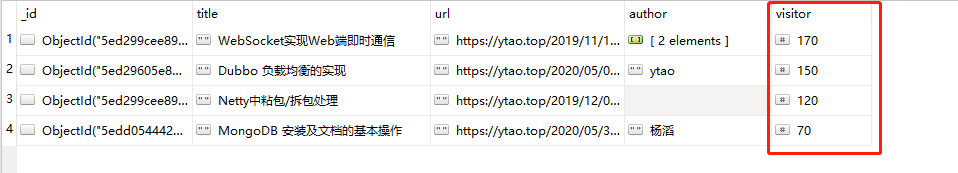## 分页操作

``````db.collection.aggregate([
{\$skip: <跳过的文档数量>},
{\$limit: <返回的文档数量>}
])
``````

``````db.article.aggregate([
{\$skip: 2},
{\$limit: 2}
])
``````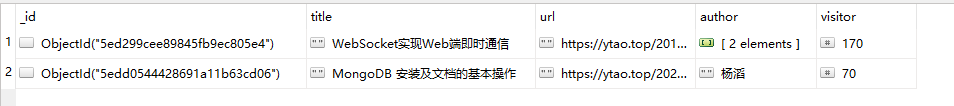## 统计文档数量

\$count用来统计文档数量，进行条件筛选时。

``````db.collection.aggregate([
{ \$count: "<显示数量的字段的名称>" }
])
``````

``````db.article.aggregate([
{ \$count: "数量" }
])
``````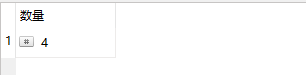## 多集合关联查询

\$lookup 是用来多集合关联查询时使用的，类似于关系型数据库中的联表查询。

``````db.collection.aggregate([
{
\$lookup: {
from: <关联的表名>,
localField: <当前表的关联字段>,
foreignField: <关联表的关联字段>,
as: <另一集合嵌入的字段名>
}
}
])
``````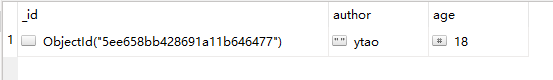``````db.article.aggregate([
{
\$lookup: {
from: "person",
localField: "author",
foreignField: "author",
as: "person_info"
}
},
{
\$match:{
"person_info.age": {\$eq: 18}
}
}
])
``````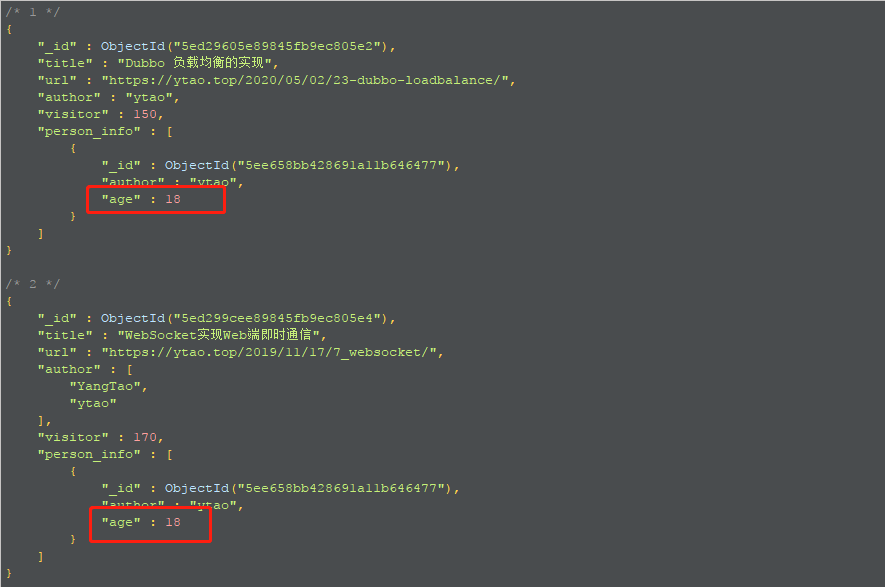# 总结

《MongoDB 安装及文档的基本操作》

《Dubbo 负载均衡的实现》

《你必须会的 JDK 动态代理和 CGLIB 动态代理》

《Redis5新特性Streams作消息队列》

0
0 收藏

### 作者的其它热门文章0 评论
0 收藏
0